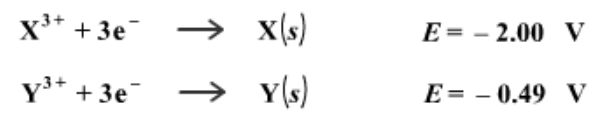# Problem: Consider these two entries from a fictional table of standard reduction potentials.What is the standard potential of a cell where X is the anode and Y is the cathode?

###### FREE Expert Solution

Calculate E°cell.

X3+(aq) + 3 e- → X(s)                       E°cell = –2.00 V                    anode

Y3+(aq) + 3 e- → Y(s)                     E°cell = – 0.49 V                    cathode

$\overline{){\mathbf{E}}{{\mathbf{°}}}_{{\mathbf{cell}}}{\mathbf{=}}{\mathbf{E}}{{\mathbf{°}}}_{{\mathbf{cathode}}}{\mathbf{-}}{\mathbf{E}}{{\mathbf{°}}}_{{\mathbf{anode}}}}$

96% (383 ratings)###### Problem Details

Consider these two entries from a fictional table of standard reduction potentials.What is the standard potential of a cell where X is the anode and Y is the cathode?